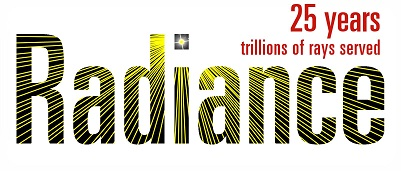# Adding colour to gray BSDFs

Dear all,

I am wondering if I missed out something about the application of the BSDF and aBSDF models in Radiance. I have a photometric BSDF that was generated from measurements by pabopto2bsdf, and a colour measurement (D65/2° XYZ and Radiance RGB). Now I would like to add the colour information to my existing BSDF model, knowing that this is a simplification since the colour probably is a function of directions.

I know that I can add a (coloured) diffuse reflection component by three real numbers in the BSDF definition. However, wouldn’t this effectively increase the reflectance unless I can subtract this component from the reflection returned by the tabular model? Or is this done internally? I was going through m_bsdf.c, but did not find the answer that is probably hidden there.

Another way, assuming that the colour of highlights is modulated by the surface, would be to multiply the BSDF by the normalised colour values. This, however, is not possible since colorfunc etc. only affect the diffuse component.

Finally, one might - under the same assumption of coloured high-lights - regenerate the BSDF (in my case from measurements) scaling the photometric values with the XYZ. Is this reasonable?

Best, Lars.

Hi Lars,

If you provide diffuse components to the BSDF or aBSDF types, they are additive.

If you apply a pattern (“colorfunc” or similar) to the material, they affect the diffuse reflection, and all transmission, but the non-diffuse reflection will be uncolored.

If you have a tensor tree BSDF, it may be possible to edit the XML file directly to add the components you want. The other option would be using pabopto2xyz to combine measured values for pabopto2bsdf to obtain a colored BSDF.

Cheers,
-Greg

Hi Greg,

first, thank you for confirming these assumptions - this saved me a lot of time for test-cases!

I was wondering how to process the gray measured BSDFs with rcalc, e.g. how to scale the DSF or BSDF with the XYZ values - multiplying with XYZ [0:100] makes little sense, so would I rather multiply with the normalised xyz (x=X/(X+Y+Z),…) here?

Is there any starting point for “edit the XML file directly to add the components”? I have no reference for a colourimetric BSDF.

Best, Lars.

It’s best to convert the XYZ to Radiance RGB using xyz_rgb.cal or similar, then multiply RR, GG, B*B, where R=G=B=1 is 100%.

For a tensor tree XML file, you can create a single entry for the CIE-u and CIE-v channels to represent a constant color. For example, transmission would have the following in addition to the usual “Visible” section:

``````	<WavelengthData>
<LayerNumber>System</LayerNumber>
<Wavelength unit="Integral">CIE-u</Wavelength>
<SourceSpectrum>CIE Illuminant D65 1nm.ssp</SourceSpectrum>
<WavelengthDataBlock>
<AngleBasis>LBNL/Shirley-Chiu</AngleBasis>
<ScatteringDataType>BTDF</ScatteringDataType>
<ScatteringData>
{ 0.210526 }
</ScatteringData>
</WavelengthDataBlock>
</WavelengthData>
<WavelengthData>
<LayerNumber>System</LayerNumber>
<Wavelength unit="Integral">CIE-v</Wavelength>
<SourceSpectrum>CIE Illuminant D65 1nm.ssp</SourceSpectrum>
<WavelengthDataBlock>
<AngleBasis>LBNL/Shirley-Chiu</AngleBasis>
<ScatteringDataType>BTDF</ScatteringDataType>
<ScatteringData>
{ 0.473684 }
</ScatteringData>
</WavelengthDataBlock>
</WavelengthData>
``````

The numbers above correspond to neutral gray, but you can compute whatever (u,v) values you need from the XYZ values using the up(X,Y,Z) and vp(X,Y,Z) functions defined in cieluv.cal.

The Klems matrix uses X and Z matrices rather than (u,v).

Cheers,
-Greg

Hi Greg,

thank you for the example, that was exactly the missing piece of the puzzle. However, it is not exactly the effect I was expecting. Is my observation correct that the given colour only modulates the contribution by “strong” light sources, but not e.g. by glow or ambient illumination? I see the color where the surface is directly illuminated by a light, but all other surfaces (that are only illuminated by indirect-diffuse reflection) appear gray. Introducing a glow source has a similar effect.

Best, Lars.

It should modulate all transmitted components equally in this example. You would have to add similar (u,v) coordinates for the other directions, including reverse transmission if present and both reflecting hemispheres.

Assuming you did all that, you might not see many indirect effects if your BSDF doesn’t have a significant Lambertian component. It depends on the distribution.